# Let Yı, Y2, ...,Yn be an iid sample from a population distribution described by the pdf...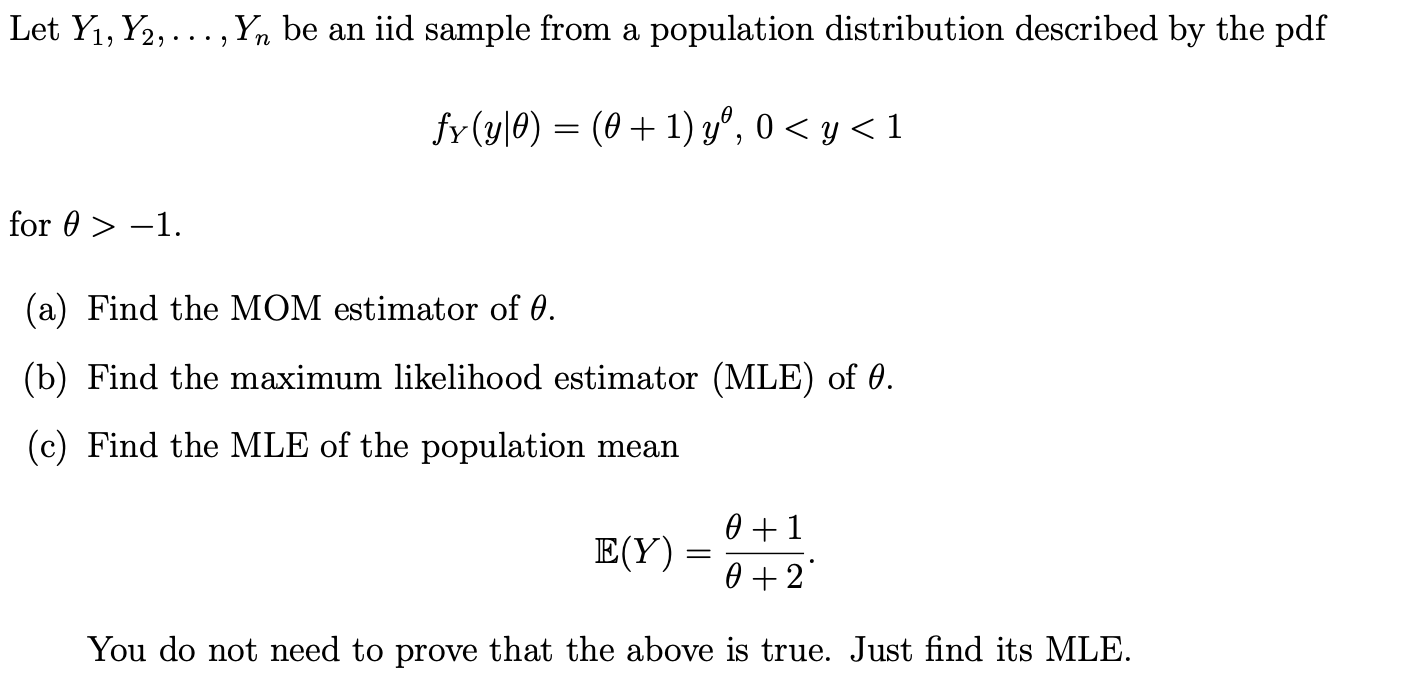Let Yı, Y2, ...,Yn be an iid sample from a population distribution described by the pdf fy(y|0) = (@+ 1) yº, o<y<1 for 0> - -1. (a) Find the MOM estimator of 0. (b) Find the maximum likelihood estimator (MLE) of 0. (c) Find the MLE of the population mean E(Y) = 0 +1 0 + 2 You do not need to prove that the above is true. Just find its MLE.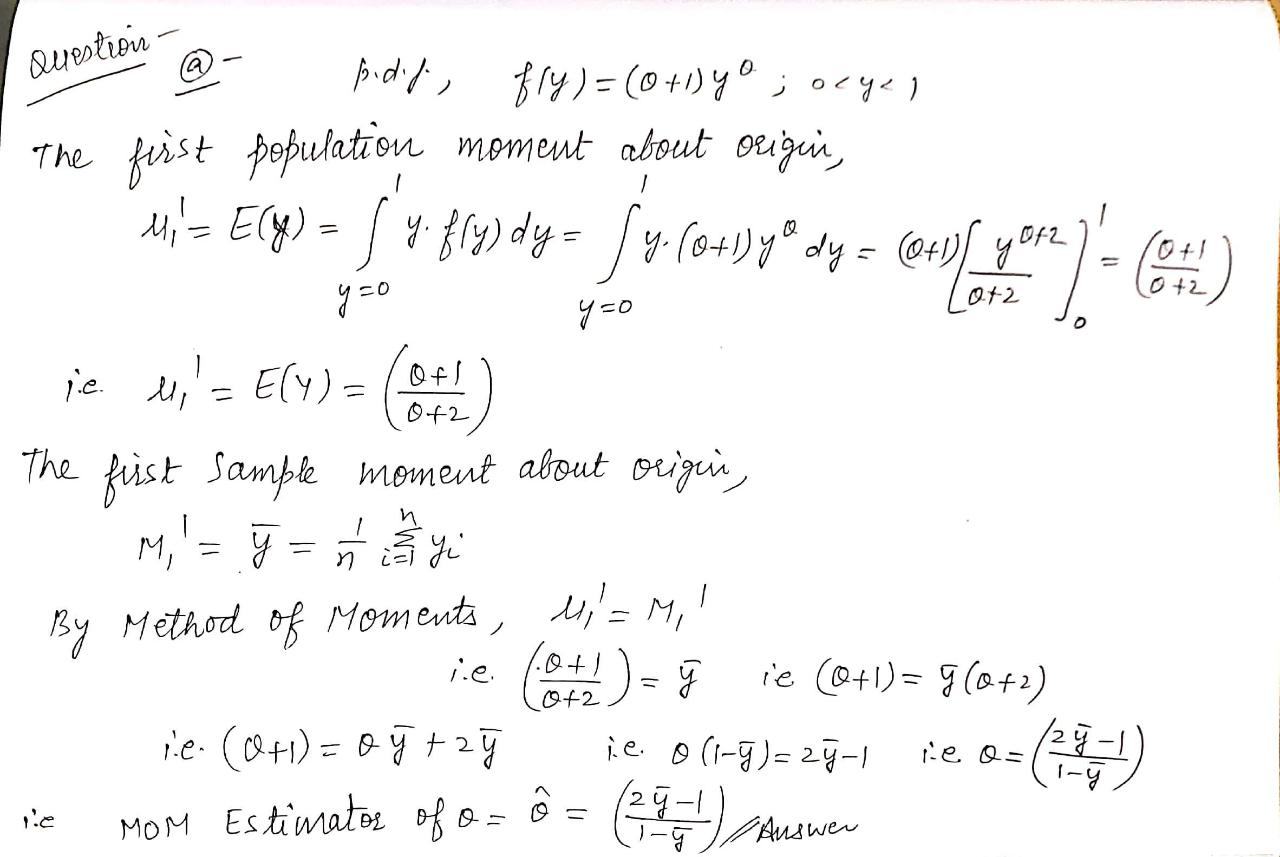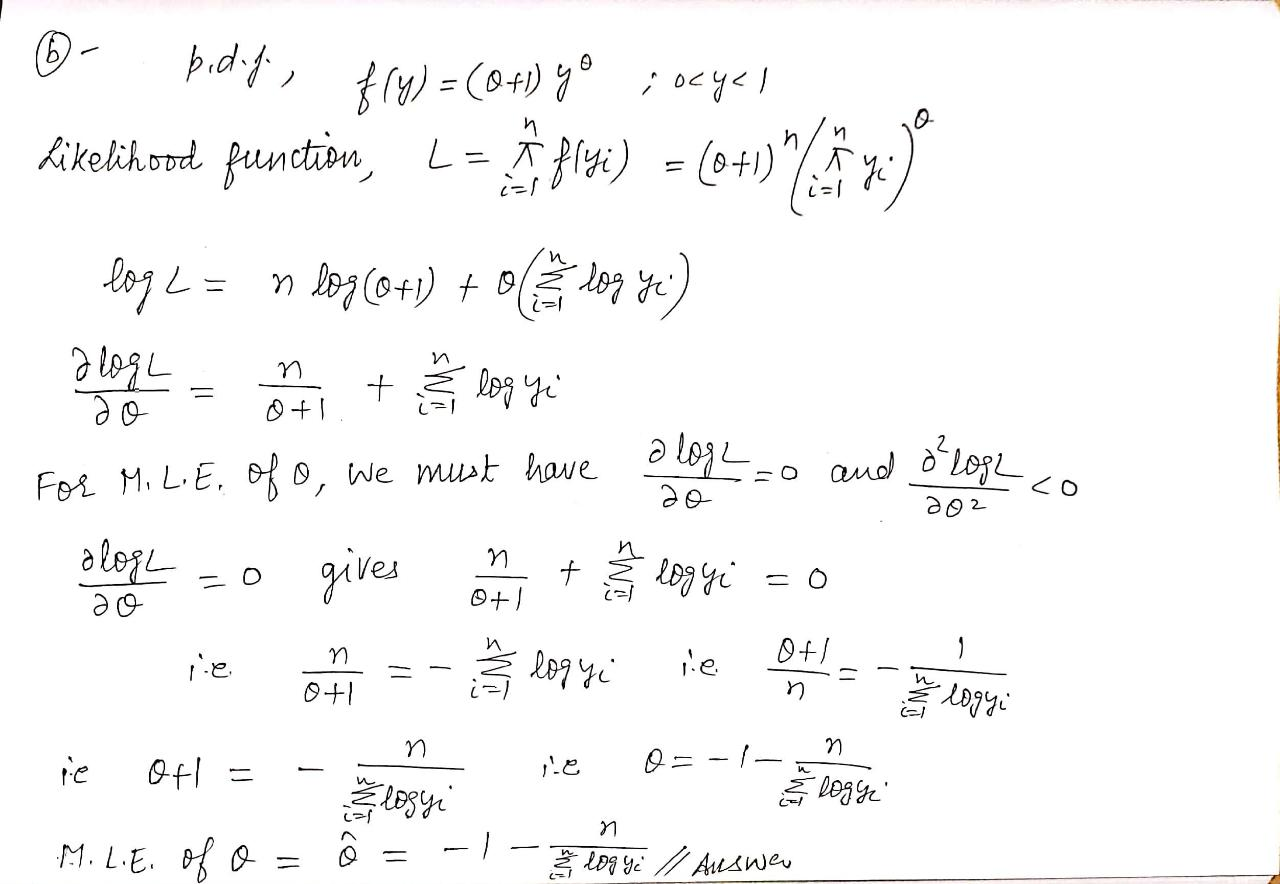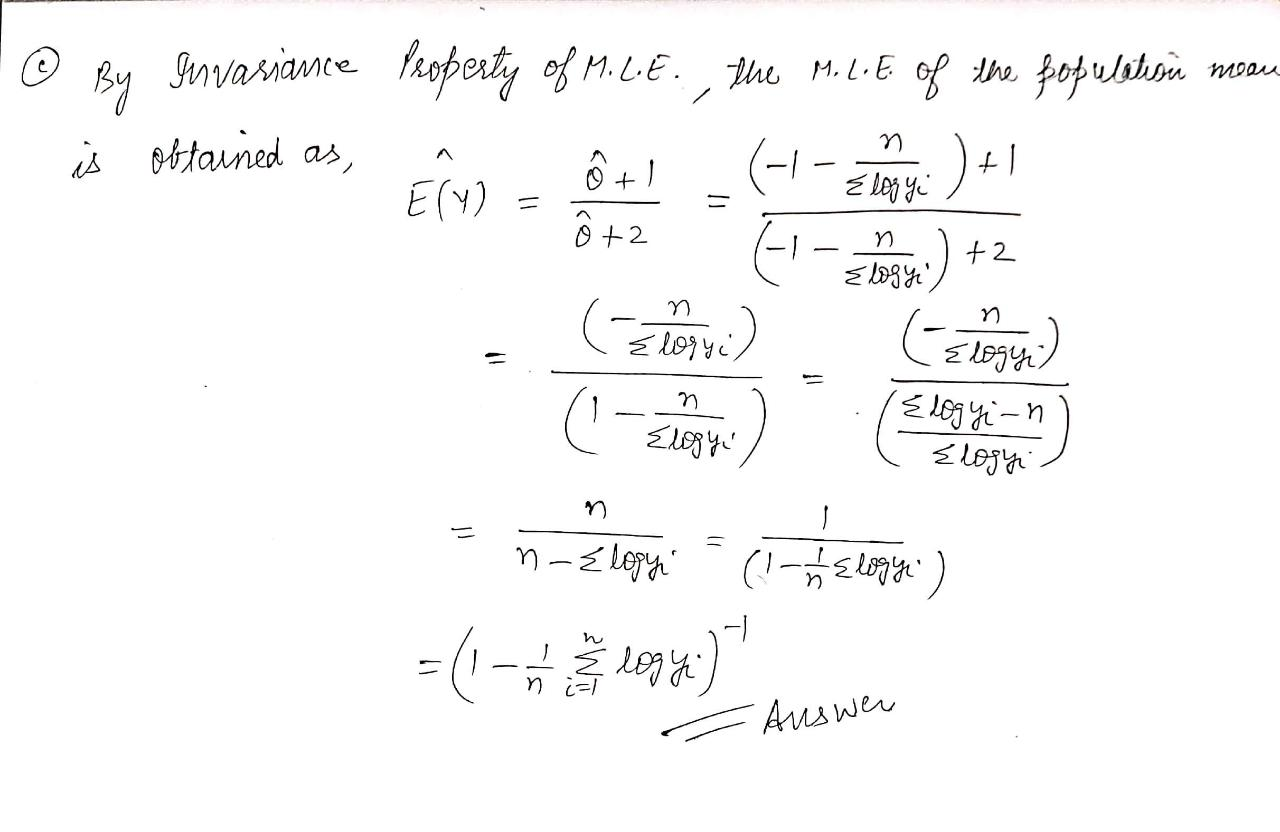##### Add Answer of: Let Yı, Y2, ...,Yn be an iid sample from a population distribution described by the pdf...
Similar Homework Help Questions
• ### Suppose Y1, Y2, ..., Yn is an iid sample from a Pareto population distribution described by...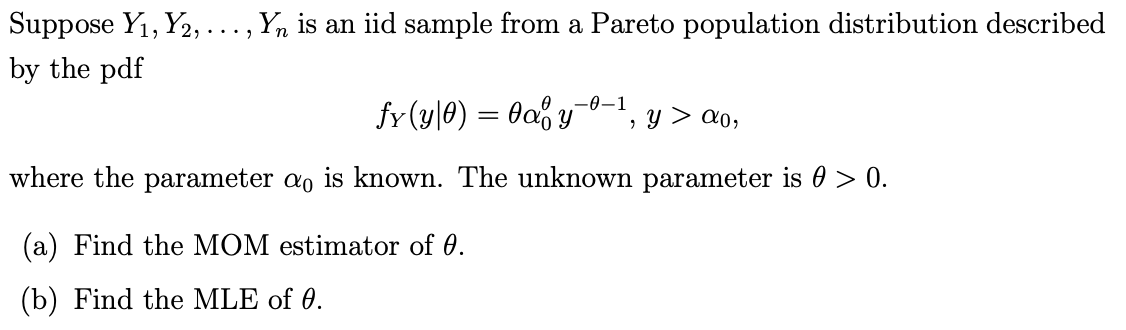Suppose Y1, Y2, ..., Yn is an iid sample from a Pareto population distribution described by the pdf fy(y|0) = 4ao y -0-1 y > 20, 2 where the parameter do is known. The unknown parameter is 0 > 0. (a) Find the MOM estimator of 0. (b) Find the MLE of 0.

• ### Let Yı,Y2, ..., Yn be iid from a population following the shifted exponential distribution with scale...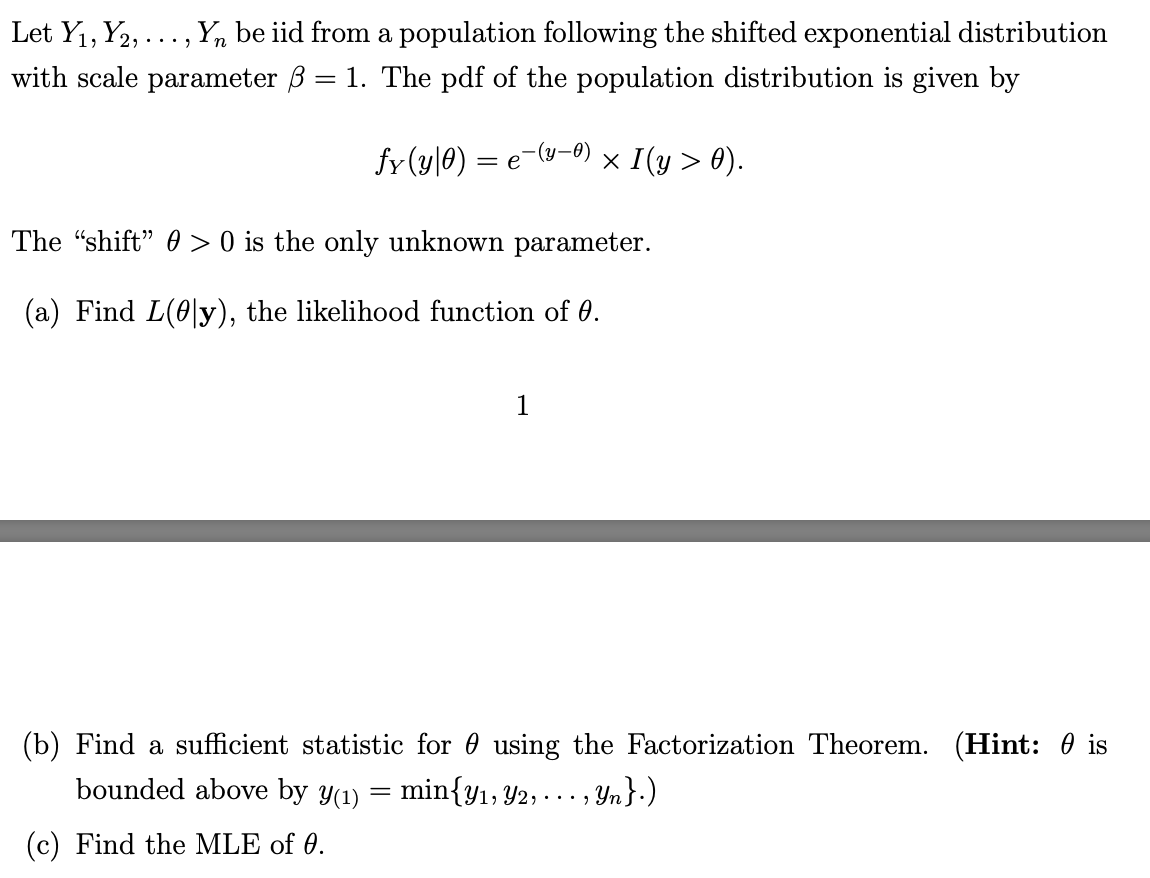Let Yı,Y2, ..., Yn be iid from a population following the shifted exponential distribution with scale parameter B = 1. The pdf of the population distribution is given by fy(y\0) = y-0) = e x I(y > 0). The "shift" @ > 0 is the only unknown parameter. (a) Find L(@ly), the likelihood function of 0. (b) Find a sufficient statistic for 0 using the Factorization Theorem. (Hint: O is bounded above by y(1) min{Y1, 42, ..., .., Yn}.) (c)...

• ### 7. Let Y1, ...,Yn be a random sample from the population with pdf f(316) = he=1/0,...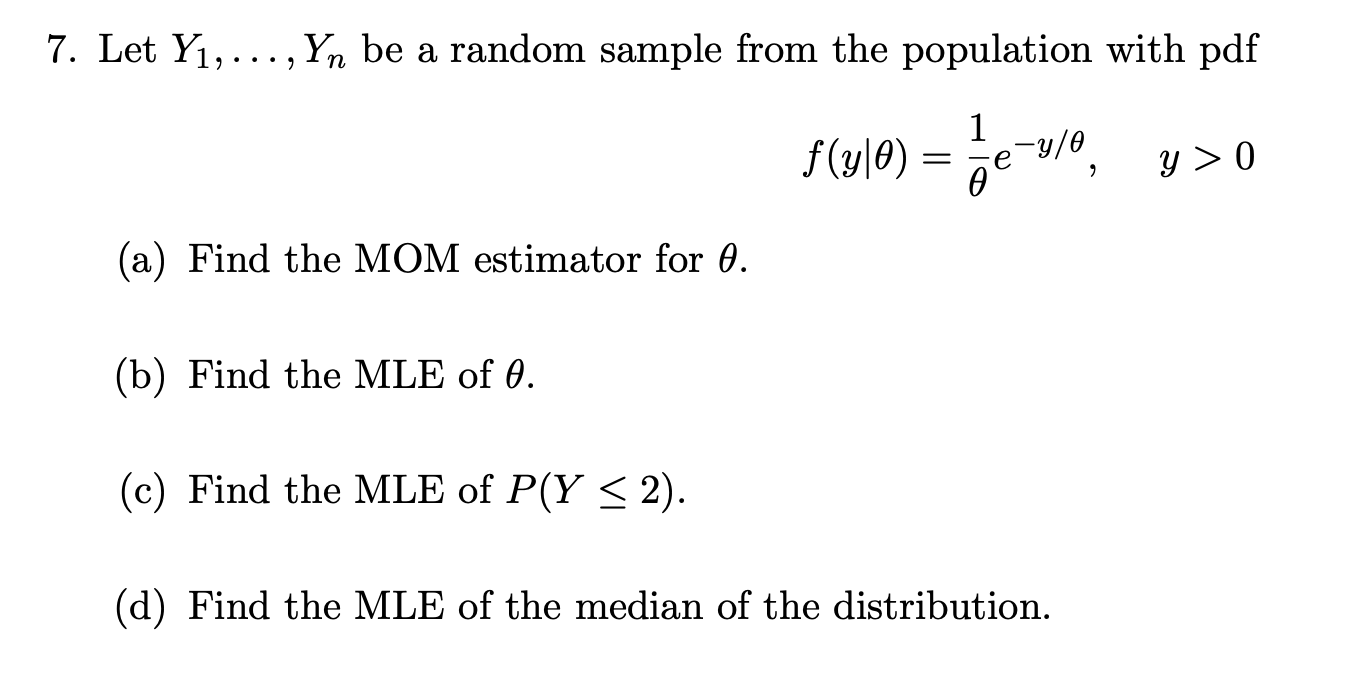7. Let Y1, ...,Yn be a random sample from the population with pdf f(316) = he=1/0, y>0 (a) Find the MOM estimator for 0. (b) Find the MLE of 0. (c) Find the MLE of P(Y < 2). (d) Find the MLE of the median of the distribution.

• ### (1 point) Let Yı, Y2, ..., Yn be a random sample from the probability density function...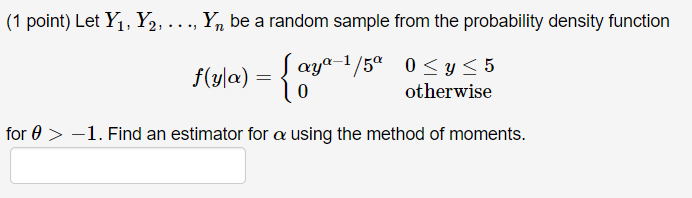(1 point) Let Yı, Y2, ..., Yn be a random sample from the probability density function f(yla) = |aya-2/5° f(y ) 0 <y< 5 otherwise 0 for > -1. Find an estimator for a using the method of moments.

• ### 2. Let Yı, ..., Yn be a random sample from an Exponential distribution with density function...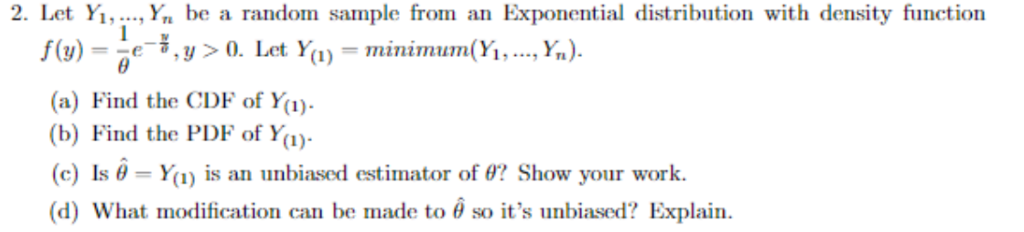2. Let Yı, ..., Yn be a random sample from an Exponential distribution with density function e-, y > 0. Let Y(1) minimum(Yi, , Yn). (a) Find the CDE of Y) b) Find the PDF of Y (c) Is θ-Yu) is an unbiased estimator of θ? Show your work. (d) what modification can be made to θ so it's unbiased? Explain.

• ### iid Let Yı, Y2, ..., Yn N(u,), where the population mean y and population variance o...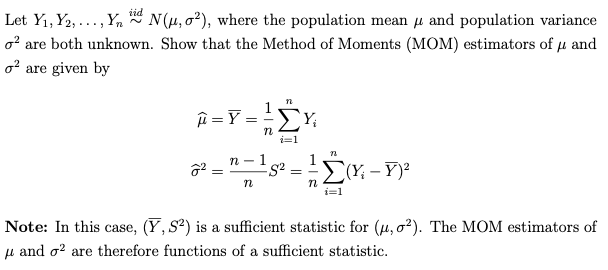iid Let Yı, Y2, ..., Yn N(u,), where the population mean y and population variance o are both unknown. Show that the Method of Moments (MOM) estimators of u and o? are given by n i =Y, Y n =1 72 = n-1 S2 (Y; -Y) n n i=1 Note: In this case, (Y, S?) is a sufficient statistic for (u, o?). The MOM estimators of u and o2 are therefore functions of a sufficient statistic.

• ### 1. Let Yı,Y2,..., Yn denote a random sample from a population with mean E (-0,) and...1. Let Yı,Y2,..., Yn denote a random sample from a population with mean E (-0,) and variance o2 € (0,0). Let Yn = n- Y. Recall that, by the law of large numbers, Yn is a consistent estimator of . (a) (10 points) Prove that Un="in is a consistent estimator of . (b) (5 points) Prove that Vn = Yn-n is not a consistent estimator of (c) (5 points) Suppose that, for each i, P(Y, - of ? Prove what...

• ### Let Yı, Y2, ..., Yn iid N (4,02), where the population mean and population variance o2...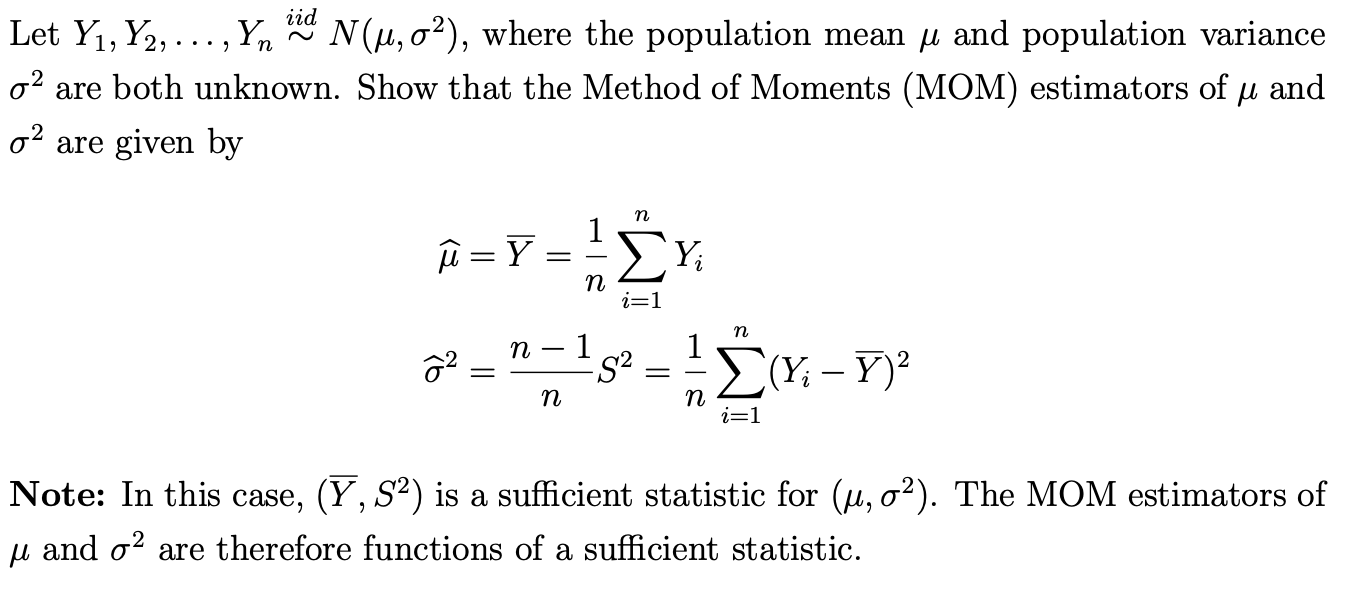Let Yı, Y2, ..., Yn iid N (4,02), where the population mean and population variance o2 are both unknown. Show that the Method of Moments (MOM) estimators of u and o2 are given by n û =Ý ΣΥ, n i=1 п n - - 1 ô2 - = s2 Ü(Y; – 7) n п i=1 Note: In this case, (Y, S2) is a sufficient statistic for (u, 02). The MOM estimators of u and o2 are therefore functions of a...

• ### Suppose that X1, X2,....Xn is an iid sample of size n from a Pareto pdf of...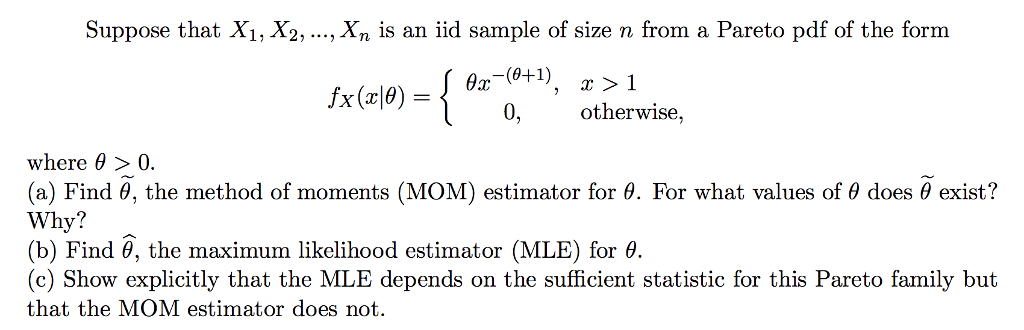Suppose that X1, X2,....Xn is an iid sample of size n from a Pareto pdf of the form 0-1) otherwise, where θ > 0. (a) Find θ the method of moments (MOM) estimator for θ For what values of θ does θ exist? Why? (b) Find θ, the maximum likelihood estimator (MLE) for θ. (c) Show explicitly that the MLE depends on the sufficient statistic for this Pareto family but that the MOM estimator does not

• ### 1. Suppose Yi,½, , Yn is an iid sample from a Bernoulli(p) population distribution, where 0< p<1 is unknown. The population pmf is py(ulp) otherwise 0, (a) Prove that Y is the maximum likelihoo...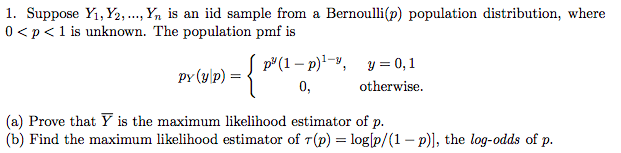1. Suppose Yi,½, , Yn is an iid sample from a Bernoulli(p) population distribution, where 0< p<1 is unknown. The population pmf is py(ulp) otherwise 0, (a) Prove that Y is the maximum likelihood estimator of p. (b) Find the maximum likelihood estimator of T(p)-loglp/(1 - p)], the log-odds of p. 1. Suppose Yi,½, , Yn is an iid sample from a Bernoulli(p) population distribution, where 0

Free Homework App